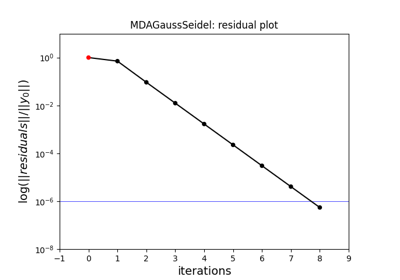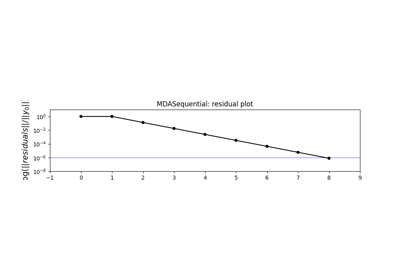gemseo / mda

# gauss_seidel module¶

A Gauss Seidel algorithm for solving MDAs.

class gemseo.mda.gauss_seidel.MDAGaussSeidel(disciplines, name=None, max_mda_iter=10, grammar_type=GrammarType.JSON, tolerance=1e-06, linear_solver_tolerance=1e-12, warm_start=False, use_lu_fact=False, over_relax_factor=None, coupling_structure=None, log_convergence=False, linear_solver='DEFAULT', linear_solver_options=None, acceleration_method=AccelerationMethod.NONE, over_relaxation_factor=1.0)[source]

Bases: MDA

Perform an MDA using the Gauss-Seidel algorithm.

This algorithm is a fixed point iteration method to solve systems of non-linear equations of the form,

$\begin{split}\left\{ \begin{matrix} F_1(x_1, x_2, \dots, x_n) = 0 \\ F_2(x_1, x_2, \dots, x_n) = 0 \\ \vdots \\ F_n(x_1, x_2, \dots, x_n) = 0 \end{matrix} \right.\end{split}$

Beginning with $$x_1^{(0)}, \dots, x_n^{(0)}$$, the iterates are obtained by performing sequentially the following $$n$$ steps.

Step 1: knowing $$x_2^{(i)}, \dots, x_n^{(i)}$$, compute $$x_1^{(i+1)}$$ by solving,

$r_1\left( x_1^{(i+1)} \right) = F_1(x_1^{(i+1)}, x_2^{(i)}, \dots, x_n^{(i)}) = 0.$

Step $$k \leq n$$: knowing $$x_1^{(i+1)}, \dots, x_{k-1}^{(i+1)}$$ on one hand, and $$x_{k+1}^{(i)}, \dots, x_n^{(i)}$$ on the other hand, compute $$x_1^{(i+1)}$$ by solving,

$r_k\left( x_k^{(i+1)} \right) = F_1(x_1^{(i+1)}, \dots, x_{k-1}^{(i+1)}, x_k^{(i+1)}, x_{k+1}^{(i)}, \dots, x_n^{(i)}) = 0.$

These $$n$$ steps account for one iteration of the Gauss-Seidel method.

Initialize self. See help(type(self)) for accurate signature.

Parameters:
• disciplines (Sequence[MDODiscipline]) – The disciplines from which to compute the MDA.

• name (str | None) – The name to be given to the MDA. If None, use the name of the class.

• max_mda_iter (int) –

The maximum iterations number for the MDA algorithm.

By default it is set to 10.

• grammar_type (MDODiscipline.GrammarType) –

The type of the input and output grammars.

By default it is set to “JSONGrammar”.

• tolerance (float) –

The tolerance of the iterative direct coupling solver; the norm of the current residuals divided by initial residuals norm shall be lower than the tolerance to stop iterating.

By default it is set to 1e-06.

• linear_solver_tolerance (float) –

The tolerance of the linear solver in the adjoint equation.

By default it is set to 1e-12.

• warm_start (bool) –

Whether the second iteration and ongoing start from the previous coupling solution.

By default it is set to False.

• use_lu_fact (bool) –

Whether to store a LU factorization of the matrix when using adjoint/forward differentiation. to solve faster multiple RHS problem.

By default it is set to False.

• over_relax_factor (float | None) – Deprecated, please consider using MDA.over_relaxation_factor instead. The relaxation coefficient, used to make the method more robust, if 0<over_relax_factor<1 or faster if 1<over_relax_factor<=2. If over_relax_factor =1., it is deactivated.

• coupling_structure (MDOCouplingStructure | None) – The coupling structure to be used by the MDA. If None, it is created from disciplines.

• log_convergence (bool) –

Whether to log the MDA convergence, expressed in terms of normed residuals.

By default it is set to False.

• linear_solver (str) –

The name of the linear solver.

By default it is set to “DEFAULT”.

• linear_solver_options (Mapping[str, Any] | None) – The options passed to the linear solver factory.

• acceleration_method (AccelerationMethod) –

The acceleration method to be used to improve the convergence rate of the fixed point iteration method.

By default it is set to “NoTransformation”.

• over_relaxation_factor (float) –

The over-relaxation factor.

By default it is set to 1.0.

all_couplings: list[str]

The names of the coupling variables.

assembly: JacobianAssembly
cache: AbstractCache | None

The cache containing one or several executions of the discipline according to the cache policy.

coupling_structure: MDOCouplingStructure

The coupling structure to be used by the MDA.

data_processor: DataProcessor

A tool to pre- and post-process discipline data.

exec_for_lin: bool

Whether the last execution was due to a linearization.

input_grammar: BaseGrammar

The input grammar.

jac: dict[str, dict[str, ndarray]]

The Jacobians of the outputs wrt inputs.

The structure is {output: {input: matrix}}.

lin_cache_tol_fact: float

The tolerance factor to cache the Jacobian.

linear_solver: str

The name of the linear solver.

linear_solver_options: Mapping[str, Any]

The options of the linear solver.

linear_solver_tolerance: float

The tolerance of the linear solver in the adjoint equation.

matrix_type: JacobianAssembly.JacobianType

The type of the matrix.

name: str

The name of the discipline.

norm0: float | None

The reference residual, if any.

normed_residual: float

The normed residual.

output_grammar: BaseGrammar

The output grammar.

property over_relax_factor: float

The over-relaxation factor.

re_exec_policy: ReExecutionPolicy

The policy to re-execute the same discipline.

reset_history_each_run: bool

Whether to reset the history of MDA residuals before each run.

residual_history: list[float]

The history of MDA residuals.

residual_variables: Mapping[str, str]

The output variables mapping to their inputs, to be considered as residuals; they shall be equal to zero.

run_solves_residuals: bool

Whether the run method shall solve the residuals.

scaling: ResidualScaling

The scaling method applied to MDA residuals for convergence monitoring.

strong_couplings: list[str]

The names of the strong coupling variables.

tolerance: float

The tolerance of the iterative direct coupling solver.

use_lu_fact: bool

Whether to store a LU factorization of the matrix.

warm_start: bool

Whether the second iteration and ongoing start from the previous solution.

## Examples using MDAGaussSeidel¶MDA

MDAGauss-Seidel MDA

Gauss-Seidel MDAHybrid Jacobi/Newton MDA

Hybrid Jacobi/Newton MDAMDA residuals

MDA residuals